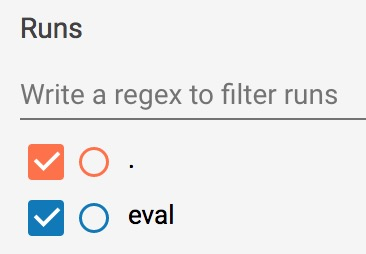TensorFlow 2.0 RC is available

# 创建自定义 Estimator

git clone https://github.com/tensorflow/models/
cd models/samples/core/get_started


python custom_estimator.py


## 预创建的 Estimator 与自定义 Estimator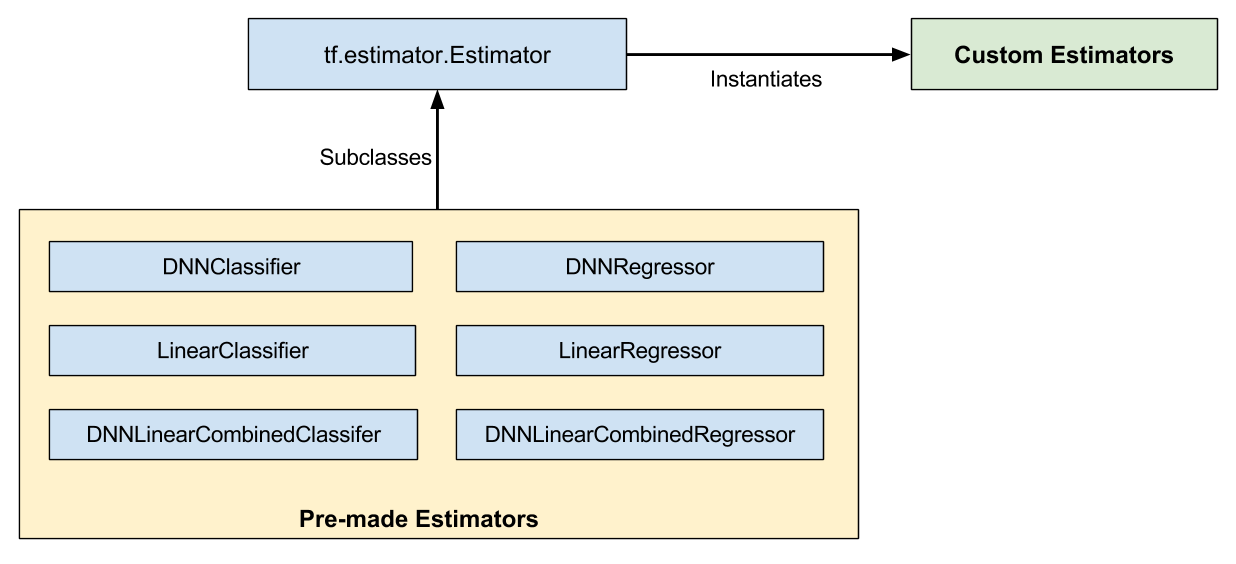• 如果采用预创建的 Estimator，则有人已为您编写了模型函数。
• 如果采用自定义 Estimator，则您必须自行编写模型函数。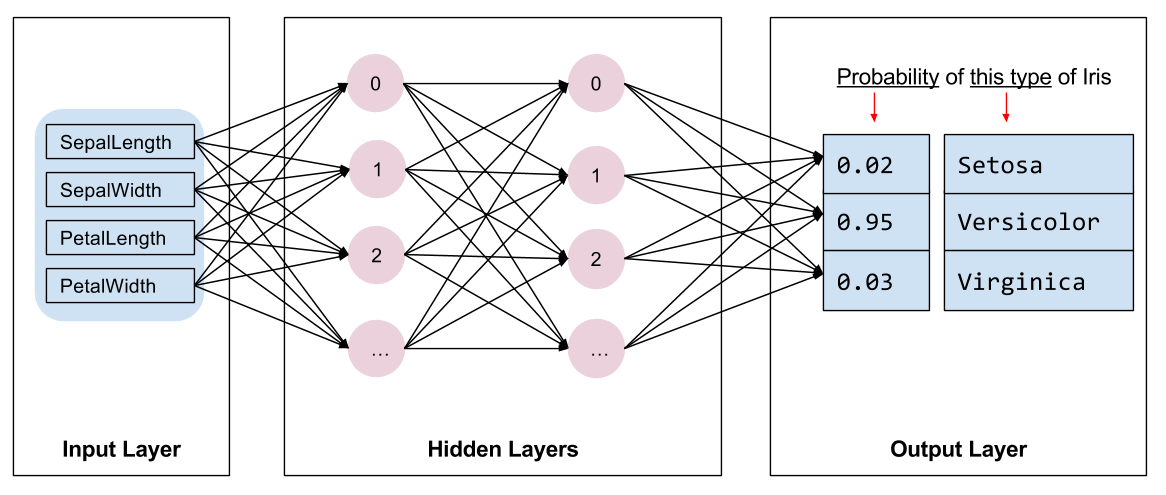## 编写输入函数

def train_input_fn(features, labels, batch_size):
"""An input function for training"""
# Convert the inputs to a Dataset.
dataset = tf.data.Dataset.from_tensor_slices((dict(features), labels))

# Shuffle, repeat, and batch the examples.
dataset = dataset.shuffle(1000).repeat().batch(batch_size)

# Return the read end of the pipeline.
return dataset.make_one_shot_iterator().get_next()


## 创建特征列

# Feature columns describe how to use the input.
my_feature_columns = []
for key in train_x.keys():
my_feature_columns.append(tf.feature_column.numeric_column(key=key))


## 编写模型函数

def my_model_fn(
features, # This is batch_features from input_fn
labels,   # This is batch_labels from input_fn
mode,     # An instance of tf.estimator.ModeKeys
params):  # Additional configuration


classifier = tf.estimator.Estimator(
model_fn=my_model,
params={
'feature_columns': my_feature_columns,
# Two hidden layers of 10 nodes each.
'hidden_units': [10, 10],
# The model must choose between 3 classes.
'n_classes': 3,
})


## 定义模型

### 定义输入层

model_fn 的第一行调用 tf.feature_column.input_layer，以将特征字典和 feature_columns 转换为模型的输入，如下所示：

    # Use input_layer to apply the feature columns.
net = tf.feature_column.input_layer(features, params['feature_columns'])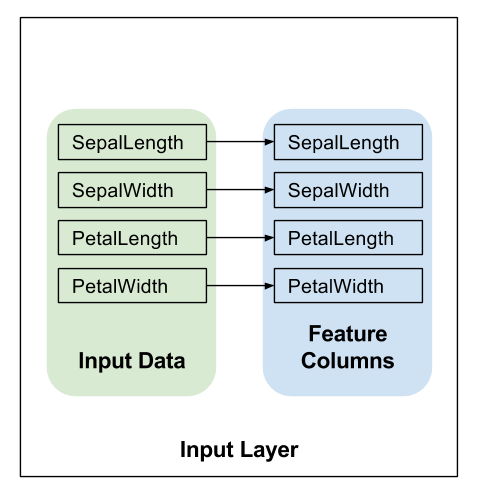### 隐藏层

    # Build the hidden layers, sized according to the 'hidden_units' param.
for units in params['hidden_units']:
net = tf.layers.dense(net, units=units, activation=tf.nn.relu)

• units 参数会定义指定层中输出神经元的数量。
• activation 参数会定义激活函数 - 在这种情况下为 Relu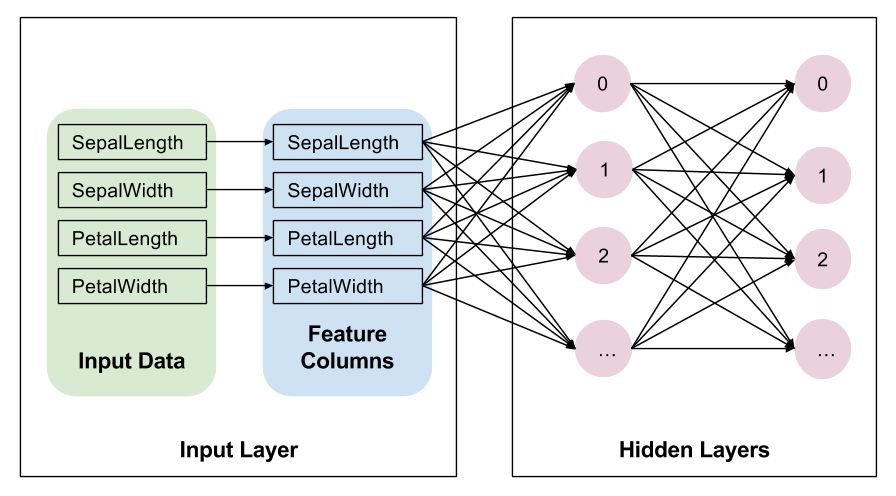### 输出层

    # Compute logits (1 per class).
logits = tf.layers.dense(net, params['n_classes'], activation=None)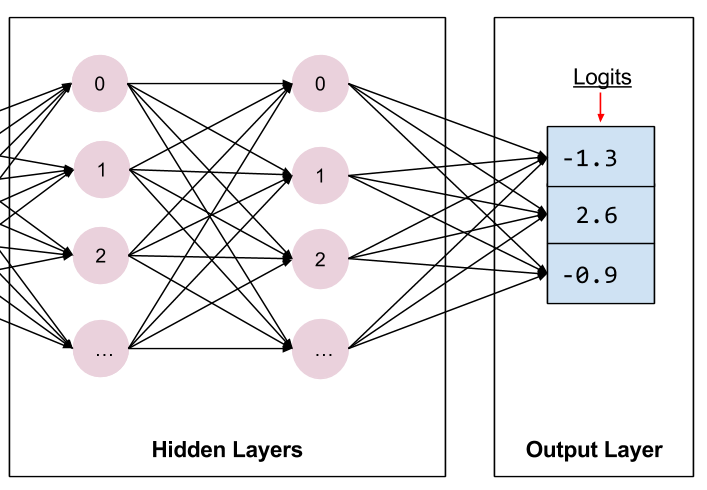## 实现训练、评估和预测

def my_model_fn(
features, # This is batch_features from input_fn
labels,   # This is batch_labels from input_fn
mode,     # An instance of tf.estimator.ModeKeys, see below
params):  # Additional configuration


Estimator 方法 Estimator 模式
train() ModeKeys.TRAIN
evaluate() ModeKeys.EVAL
predict() ModeKeys.PREDICT

classifier = tf.estimator.Estimator(...)
classifier.train(input_fn=lambda: my_input_fn(FILE_TRAIN, True, 500))


### 预测

# Compute predictions.
predicted_classes = tf.argmax(logits, 1)
if mode == tf.estimator.ModeKeys.PREDICT:
predictions = {
'class_ids': predicted_classes[:, tf.newaxis],
'probabilities': tf.nn.softmax(logits),
'logits': logits,
}
return tf.estimator.EstimatorSpec(mode, predictions=predictions)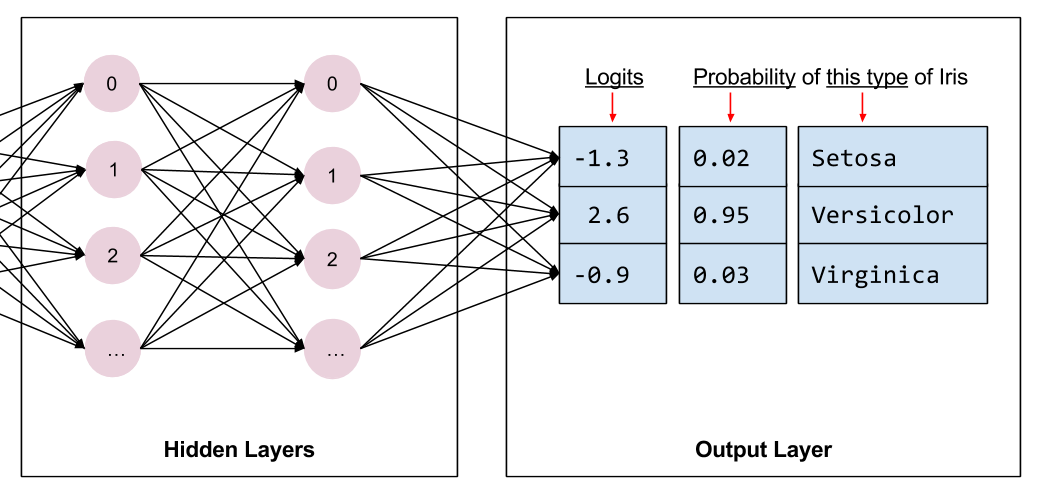predictions 存储的是下列三个键值对：

• class_ids 存储的是类别 ID（0、1 或 2），表示模型对此样本最有可能归属的品种做出的预测。
• probabilities 存储的是三个概率（在本例中，分别是 0.02、0.95 和 0.03）
• logit 存储的是原始对数值（在本例中，分别是 -1.3、2.6 和 -0.9）

### 计算损失

# Compute loss.
loss = tf.losses.sparse_softmax_cross_entropy(labels=labels, logits=logits)


### 评估

# Compute evaluation metrics.
accuracy = tf.metrics.accuracy(labels=labels,
predictions=predicted_classes,
name='acc_op')


• loss：这是模型的损失
• eval_metric_ops：这是可选的指标字典。

metrics = {'accuracy': accuracy}
tf.summary.scalar('accuracy', accuracy)

if mode == tf.estimator.ModeKeys.EVAL:
return tf.estimator.EstimatorSpec(
mode, loss=loss, eval_metric_ops=metrics)


tf.summary.scalar 会在 TRAINEVAL 模式下向 TensorBoard 提供准确率（后文将对此进行详细的介绍）。

### 训练

optimizer = tf.train.AdagradOptimizer(learning_rate=0.1)


minimize 方法还具有 global_step 参数。TensorFlow 使用此参数来计算已经处理过的训练步数（以了解何时结束训练）。此外，global_step 对于 TensorBoard 图能否正常运行至关重要。只需调用 tf.train.get_global_step 并将结果传递给 minimizeglobal_step 参数即可。

train_op = optimizer.minimize(loss, global_step=tf.train.get_global_step())


• loss：包含损失函数的值。
• train_op：执行训练步。

return tf.estimator.EstimatorSpec(mode, loss=loss, train_op=train_op)


## 自定义 Estimator

    # Build 2 hidden layer DNN with 10, 10 units respectively.
classifier = tf.estimator.Estimator(
model_fn=my_model,
params={
'feature_columns': my_feature_columns,
# Two hidden layers of 10 nodes each.
'hidden_units': [10, 10],
# The model must choose between 3 classes.
'n_classes': 3,
})


# Train the Model.
classifier.train(
input_fn=lambda:iris_data.train_input_fn(train_x, train_y, args.batch_size),
steps=args.train_steps)


## TensorBoard

# Replace PATH with the actual path passed as model_dir
tensorboard --logdir=PATH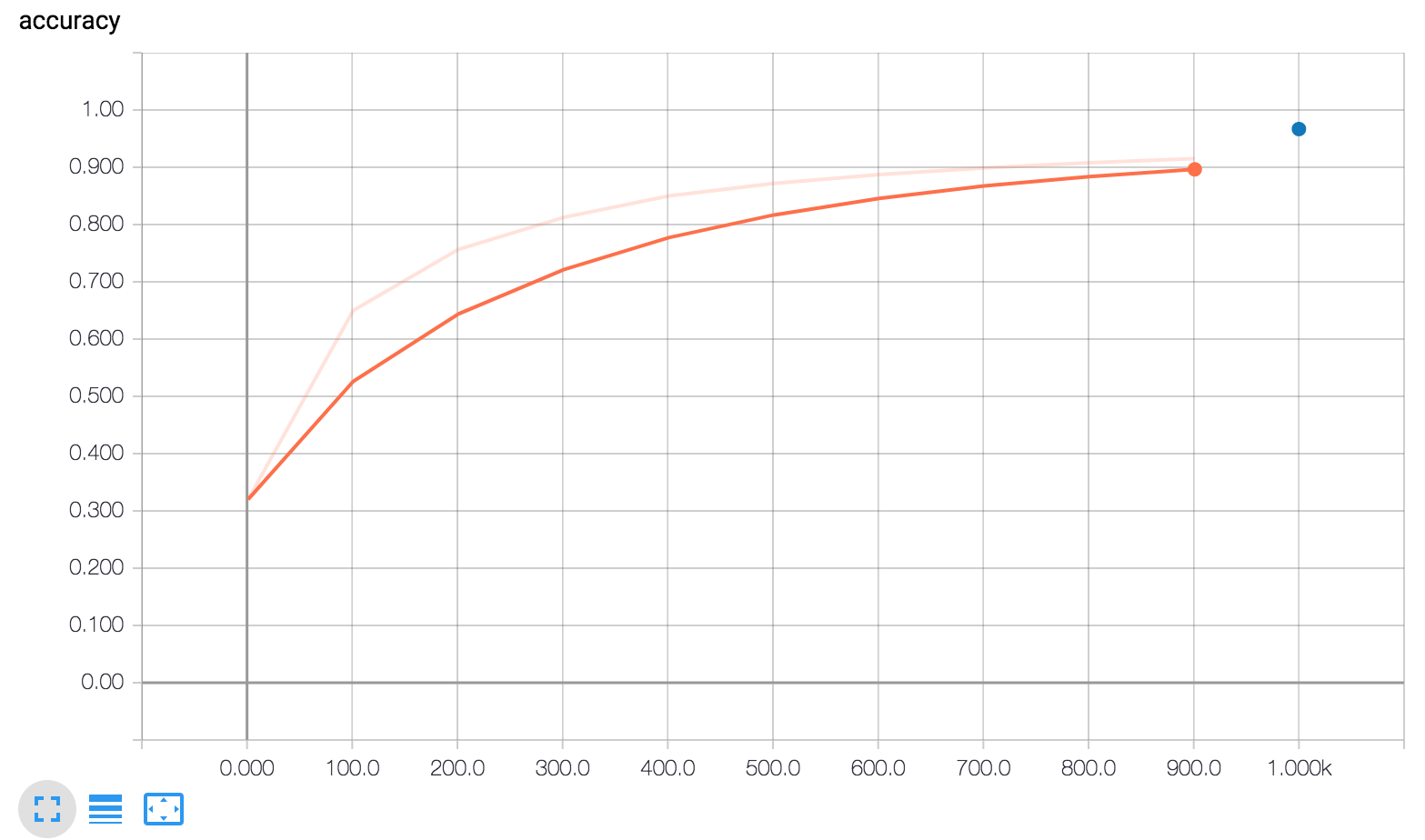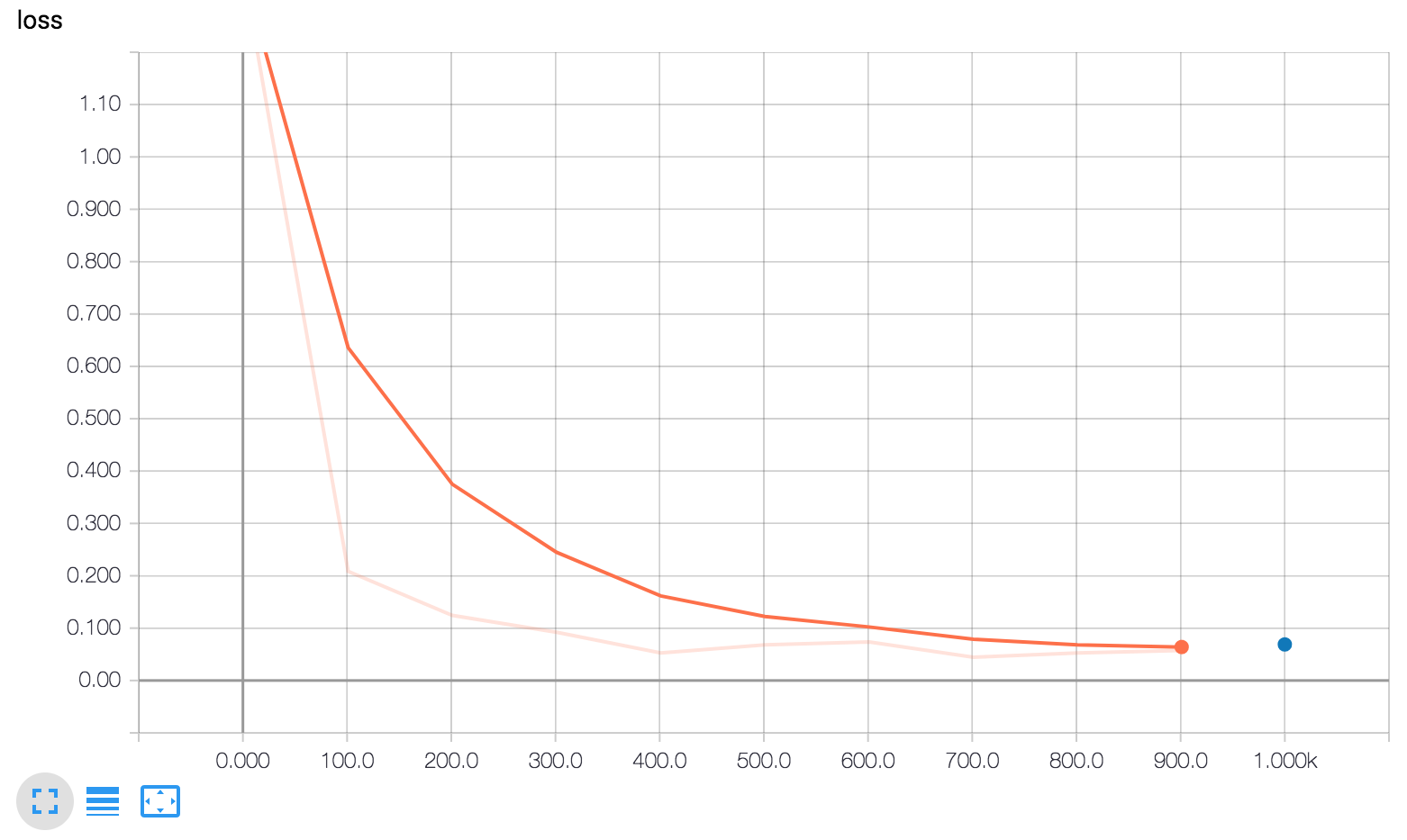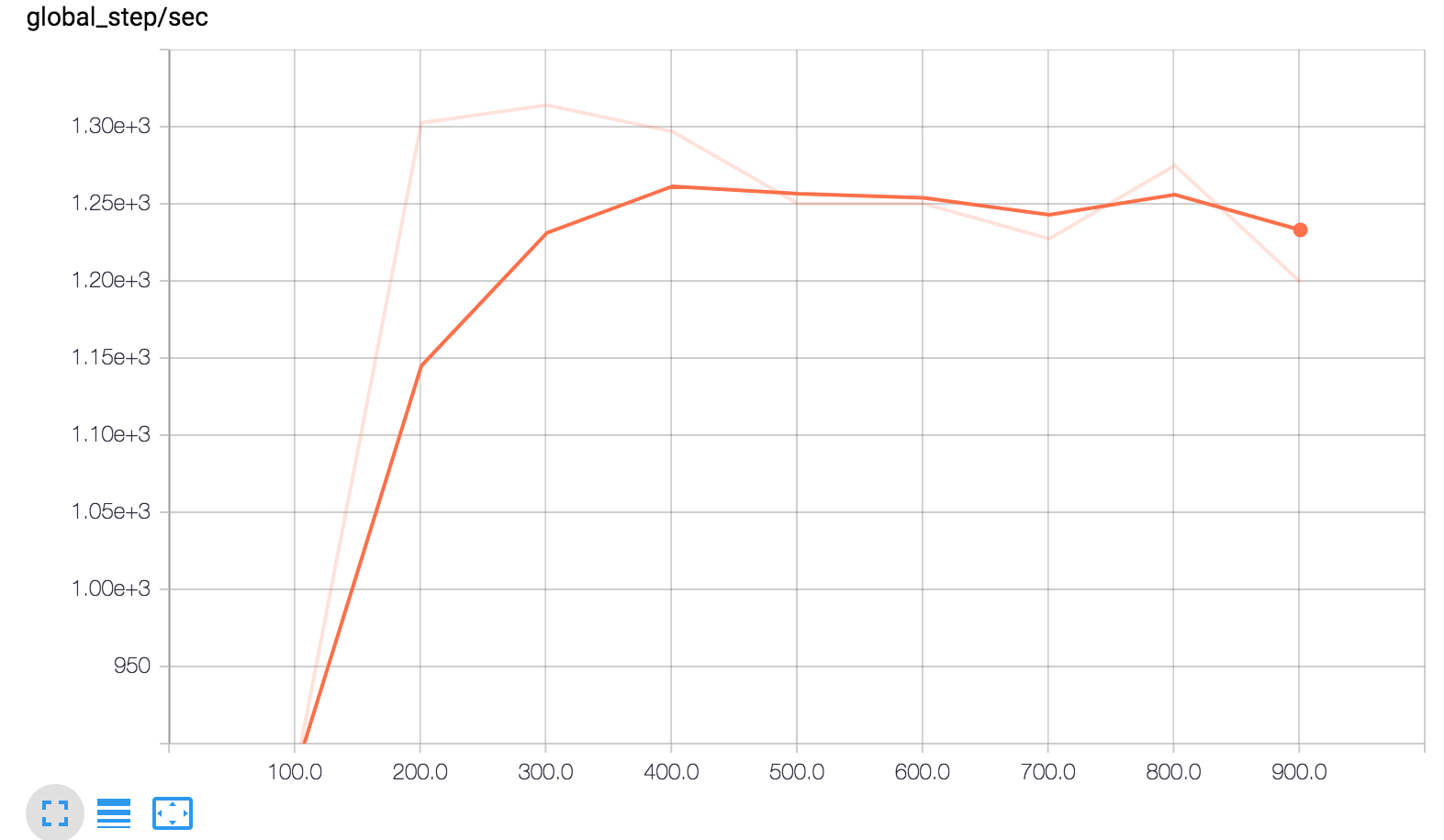TensorBoard 显示了三张图。

• global_step/sec：这是一个性能指标，显示我们在进行模型训练时每秒处理的批次数（梯度更新）。

• loss：所报告的损失。

• accuracy：准确率由下列两行记录：

• eval_metric_ops={'my_accuracy': accuracy}（评估期间）。
• tf.summary.scalar('accuracy', accuracy)（训练期间）。

• 橙线表示训练。
• 蓝点表示评估。